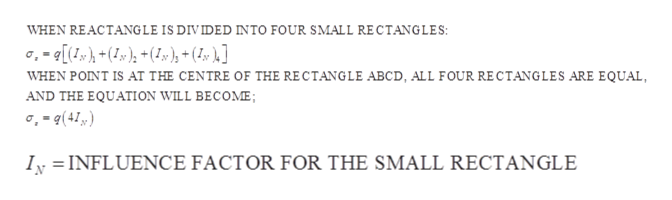# ABCD is a Raft Foundation rectangular plan with dimension of 20m*12m. The uniformly distributed load over the Raft 400kn/m².find the stress at point "O" which is the middle point of the Raft at the depth of below 6m.

Question
49 views

ABCD is a Raft Foundation rectangular plan with dimension of 20m*12m. The uniformly distributed load over the Raft 400kn/m².find the stress at point "O" which is the middle point of the Raft at the depth of below 6m.

check_circle

Step 1

Given:

Size of raft = 20m×12m

Uniform distributed load = 400 KN/m2

Depth,z=6m

Given:

Size of raft = 20m×12m

Uniform distributed load = 400 KN/m2

Depth,z=6m

Step 2

Stress at Point anywhere below the rectangular area is given by:help_outlineImage TranscriptioncloseWHEN REACTANGLE IS DIVIDED INTO FOUR SMALL RECTANGLES ,-[()(x ), + (4,y }] WHEN POINT IS AT THE CENTRE OF THE RECTANGLE ABCD, ALL FOUR RECTANGLES ARE EQUAL AND THE EQUATION WILL BECOME; ,-(4) IN INFLUENCE FACTOR FOR THE SMALL RECTANGLE fullscreen
Step 3

Now divide the rectangle ABCD into small r...

### Want to see the full answer?

See Solution

#### Want to see this answer and more?

Solutions are written by subject experts who are available 24/7. Questions are typically answered within 1 hour.*

See Solution
*Response times may vary by subject and question.
Tagged in

### Civil Engineering